# Image Approximation with Orthogonal Bases¶

Important: Please read the installation page for details about how to install the toolboxes. $\newcommand{\dotp}{\langle #1, #2 \rangle}$ $\newcommand{\enscond}{\lbrace #1, #2 \rbrace}$ $\newcommand{\pd}{ \frac{ \partial #1}{\partial #2} }$ $\newcommand{\umin}{\underset{#1}{\min}\;}$ $\newcommand{\umax}{\underset{#1}{\max}\;}$ $\newcommand{\umin}{\underset{#1}{\min}\;}$ $\newcommand{\uargmin}{\underset{#1}{argmin}\;}$ $\newcommand{\norm}{\|#1\|}$ $\newcommand{\abs}{\left|#1\right|}$ $\newcommand{\choice}{ \left\{ \begin{array}{l} #1 \end{array} \right. }$ $\newcommand{\pa}{\left(#1\right)}$ $\newcommand{\diag}{{diag}\left( #1 \right)}$ $\newcommand{\qandq}{\quad\text{and}\quad}$ $\newcommand{\qwhereq}{\quad\text{where}\quad}$ $\newcommand{\qifq}{ \quad \text{if} \quad }$ $\newcommand{\qarrq}{ \quad \Longrightarrow \quad }$ $\newcommand{\ZZ}{\mathbb{Z}}$ $\newcommand{\CC}{\mathbb{C}}$ $\newcommand{\RR}{\mathbb{R}}$ $\newcommand{\EE}{\mathbb{E}}$ $\newcommand{\Zz}{\mathcal{Z}}$ $\newcommand{\Ww}{\mathcal{W}}$ $\newcommand{\Vv}{\mathcal{V}}$ $\newcommand{\Nn}{\mathcal{N}}$ $\newcommand{\NN}{\mathcal{N}}$ $\newcommand{\Hh}{\mathcal{H}}$ $\newcommand{\Bb}{\mathcal{B}}$ $\newcommand{\Ee}{\mathcal{E}}$ $\newcommand{\Cc}{\mathcal{C}}$ $\newcommand{\Gg}{\mathcal{G}}$ $\newcommand{\Ss}{\mathcal{S}}$ $\newcommand{\Pp}{\mathcal{P}}$ $\newcommand{\Ff}{\mathcal{F}}$ $\newcommand{\Xx}{\mathcal{X}}$ $\newcommand{\Mm}{\mathcal{M}}$ $\newcommand{\Ii}{\mathcal{I}}$ $\newcommand{\Dd}{\mathcal{D}}$ $\newcommand{\Ll}{\mathcal{L}}$ $\newcommand{\Tt}{\mathcal{T}}$ $\newcommand{\si}{\sigma}$ $\newcommand{\al}{\alpha}$ $\newcommand{\la}{\lambda}$ $\newcommand{\ga}{\gamma}$ $\newcommand{\Ga}{\Gamma}$ $\newcommand{\La}{\Lambda}$ $\newcommand{\si}{\sigma}$ $\newcommand{\Si}{\Sigma}$ $\newcommand{\be}{\beta}$ $\newcommand{\de}{\delta}$ $\newcommand{\De}{\Delta}$ $\newcommand{\phi}{\varphi}$ $\newcommand{\th}{\theta}$ $\newcommand{\om}{\omega}$ $\newcommand{\Om}{\Omega}$

This numerical tour uses several orthogonal bases to perform non-linear image approximation.

In :
options(warn=-1) # turns off warnings, to turn on: "options(warn=0)"

library(imager)
library(png)

for (f in list.files(path="nt_toolbox/toolbox_general/", pattern="*.R")) {
source(paste("nt_toolbox/toolbox_general/", f, sep=""))
}

for (f in list.files(path="nt_toolbox/toolbox_signal/", pattern="*.R")) {
source(paste("nt_toolbox/toolbox_signal/", f, sep=""))
}
options(repr.plot.width=3.5, repr.plot.height=3.5)


## Best $M$-terms Non-linear Approximation¶

This tours makes use of an orthogonal base $\Bb = \{ \psi_m \}_{m=0}^{N-1}$ of the space $\RR^N$ of the images with $N$ pixels.

The best $M$-term approximation of $f$ is obtained by a non-linear thresholding

$$f_M = \sum_{ \abs{\dotp{f}{\psi_m}}>T } \dotp{f}{\psi_m} \psi_m,$$

where the value of $T>0$ should be carefully selected so that only $M$ coefficients are not thresholded, i.e.

$$\abs{ \enscond{m}{ \abs{\dotp{f}{\psi_m}}>T } } = M.$$

The goal is to use an ortho-basis $\Bb$ so that the error $\norm{f-f_M}$ decays as fast as possible when $M$ increases, for a large class of images.

This tour studies several different orthogonal bases: Fourier, wavelets (which is at the heart of JPEG-2000), cosine, local cosine (which is at the heart of JPEG).

First we load an image of $N = n \times n$ pixels.

In :
n <- 512


Display it.

In :
imageplot(f)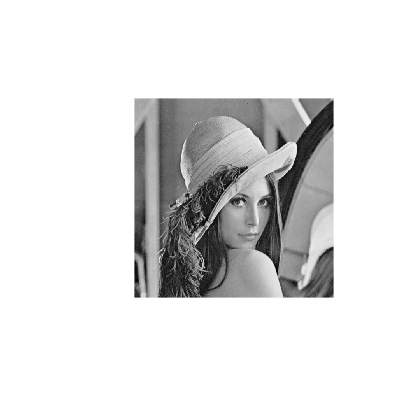## Fourier Approximation¶

The discrete 2-D Fourier atoms are defined as: $$\psi_m(x) = \frac{1}{\sqrt{N}} e^{ \frac{2i\pi}{n} ( x_1 m_1 + x_2 m_2 ) },$$ where $0 \leq m_1,m_2 < n$ indexes the frequency.

The set of inner products $\{ \dotp{f}{\psi_m} \}_m$ is computed in $O(N \log(N))$ operations with the 2-D Fast Fourier Transform (FFT) algorithm.

Compute the Fourier transform using the FFT algorithm. Note the normalization by $1/\sqrt{N}$ to ensure orthogonality (energy conservation) of the transform.

In :
fF <- fft(f)/n


Display its magnitude (in log scale). We use the pylab function fftshift to put the low frequency in the center.

In :
imageplot(log(1e-5 + abs(fftshift(as.matrix(fF)))))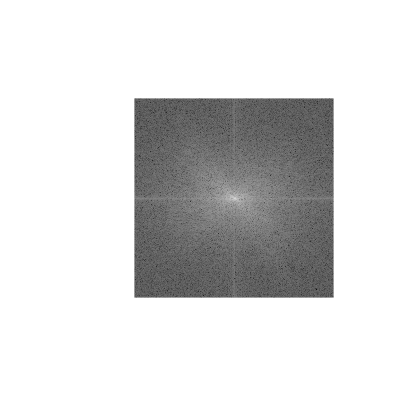An image is recovered from a set of coefficients $c_m$ using the inverse Fourier Transform that implements the formula

$$f_M = \sum_m c_m \psi_m.$$

Perform a thresholding.

In :
T <- .3
c <- fF * (abs(fF) > T)


Inverse the Fourier transform.

In :
fM <- Re( (fft(c, inverse=TRUE)/length(c))*n )


Display the approximation.

In :
imageplot(clamp(fM))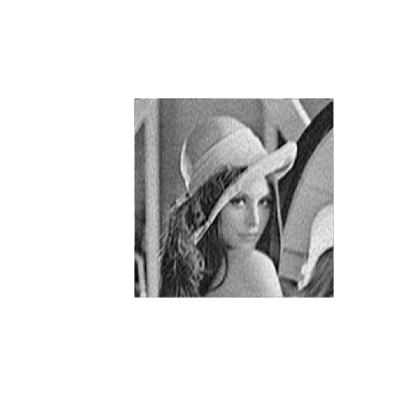Exercise 1

Compute a best $M$-term approximation in the Fourier basis of $f$, for $M \in \{N/100, N/20\}$. Compute the approximation using a well chosen hard threshold value $T$.

In :
options(repr.plot.width=7, repr.plot.height=3.5)

source("nt_solutions/coding_1_approximation/exo1.R")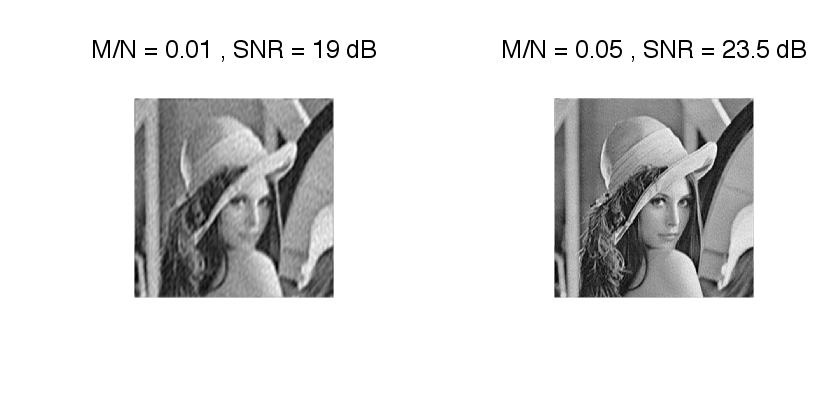In :
## Insert your code here.


The best $M$-term approximation error is computed using the conservation of energy as

$$\epsilon[M]^2 = \norm{f-f_M}^2 = \sum_{ \abs{\dotp{f}{\psi_m}} \leq T } \abs{\dotp{f}{\psi_m}}^2.$$

If one denotes by $\{ c_R[k] \}_{k=0}^{N-1}$ the set of coefficients magnitudes $\abs{\dotp{f}{\psi_m}}$ ordered by decaying magnitudes, then this error is easily computed as $$\epsilon[M]^2 = \sum_{k=M}^{N-1} c_R[k]^2 = \norm{f}^2 - \sum_{k=0}^{M-1} c_R[k]^2.$$ This means that $\epsilon^2$ is equal to $\norm{f}^2$ minus the discrete primitive of $c_R^2$.

Exercise 2

Compute and display in log scales the ordered coefficients $c_R$. Hint: a discrete primitive can be computed using the function cumsum.

In :
options(repr.plot.width=3.5, repr.plot.height=3.5)

source("nt_solutions/coding_1_approximation/exo2.R")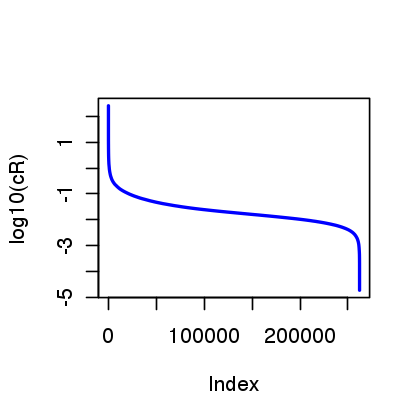In :
## Insert your code here.


Exercise 3

Compute and display in log-scale the non-linear approximation error $\epsilon[M]^2$. Store the values of $\epsilon[M]^2$ in a vector $err\_fft$.

In :
options(repr.plot.width=3.5, repr.plot.height=3.5)

source("nt_solutions/coding_1_approximation/exo3.R")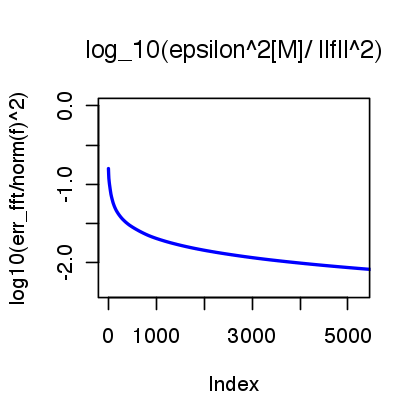In :
## Insert your code here.


## Wavelet Approximation¶

The Wavelet basis of continuous 2-D functions is defined by scaling and translating three mother atoms $\{\psi^H,\psi^V,\psi^D\}$: $$\psi_{j,n}^k(x) = \frac{1}{2^j}\psi^k\pa{\frac{x-2^j n}{2^j}}$$

Non-linear wavelet approximation is a the heart of the JPEG-2000 compression standard.

The set of inner products $\{ \dotp{f}{\psi_m} \}_m$ is computed in $O(N)$ operations with the 2-D Fast Wavelet Transform algorithm.

Perform a wavelet transform. Here we use a daubechies wavelet transform.

In :
Jmin <- 1

h <- compute_wavelet_filter("Daubechies",10)

fW <- perform_wavortho_transf(f, Jmin, + 1, h)


Display the coefficients.

In :
plot_wavelet(fW, Jmin)
title(main='Wavelet coefficients')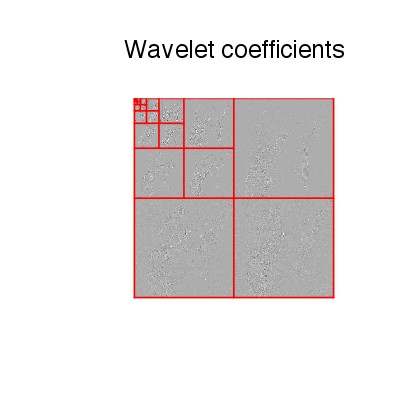Exercise 4

Compute a best $M$-term approximation in the wavelet basis of $f$, for $M \in \{N/100, N/20\}$. Compute the approximation using a well chosen hard threshold value $T$. Note that the inverse wavelet transform is obtained by replacing the +1 by a -1 in the definition of the transform.

In :
options(repr.plot.width=7, repr.plot.height=3.5)

source("nt_solutions/coding_1_approximation/exo4.R")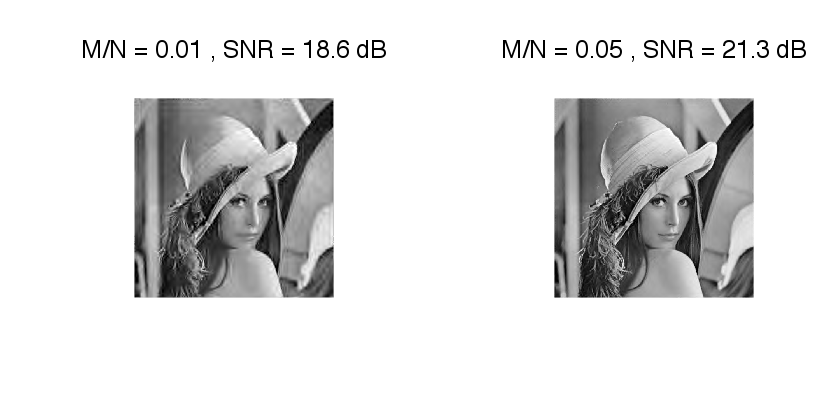In :
## Insert your code here.


Exercise 5

Compute and display in log-scale the non-linear approximation error $\epsilon[M]^2$. Compares the Fourier and wavelets approximations. Store the values of $\epsilon[M]^2$ in a vector $err\_wav$.

In :
options(repr.plot.width=4, repr.plot.height=4)

source("nt_solutions/coding_1_approximation/exo5.R")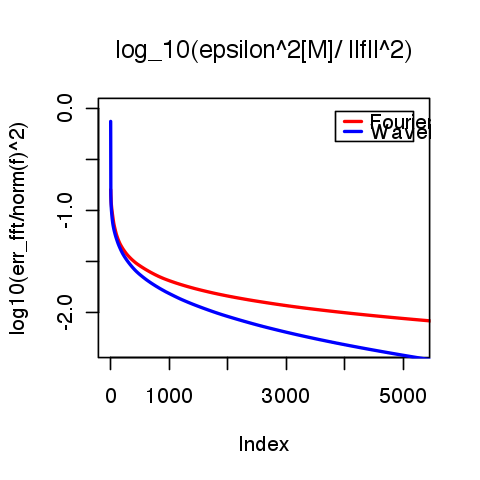In :
## Insert your code here


## Cosine Approximation¶

The discrete cosine approximation (DCT) is similar to the Fourier approximation, excepted that it used symmetric boundary condition instead of periodic boundary condition, and is thus more useful to approximate image.

A 1-D cosine atom of $n$ sample is defined as $$\bar\psi_m(x) = \frac{1}{\sqrt{N}} \cos\pa{ \frac{2\pi}{N} (x-1/2) m }$$ A 2-D cosine atom is obtained by tensor product of 1-D atoms $$\psi_{m_1,m_2}(x_1,x_2) = \bar\psi_{m_1}(x_1) \bar\psi_{m_2}(x_2).$$ On the contrary to the Fourier 2-D atoms, these 2-D DCT atoms are not oriented (they contains 4 Fourier frequencies).

The set of inner products $\{ \dotp{f}{\psi_m} \}_m$ is computed in $O(N \log(N))$ operations with the 2-D Fast Cosine Transform algorithm.

In :
dct2 <- function(f){
return( t(dct_2d(t(dct_2d(as.matrix(f))))) )
}

idct2 <- function(f){
return( t(dct_2d(t(dct_2d(as.matrix(f),inverted=T)), inverted=T)) )
}

fC <- dct2(f)

In :
imageplot(log(1e-5 + abs(fC)))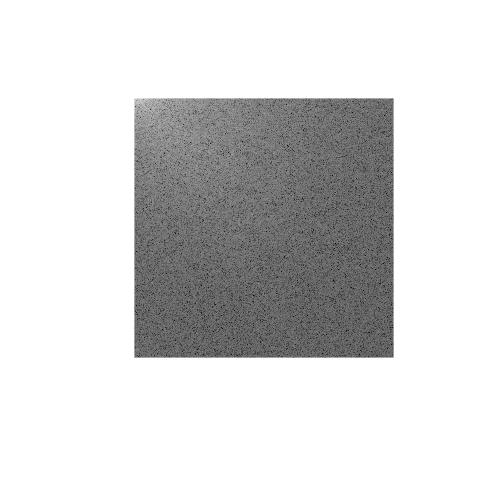Exercise 6

Compute a best $M$-term approximation in the wavelet basis of $f$, for $M \in \{N/100, N/20\}$. Compute the approximation using a well chosen hard threshold value $T$. Note that the inverse DCT transform is obtained with the function idct.

In :
options(repr.plot.width=7, repr.plot.height=3.5)

source("nt_solutions/coding_1_approximation/exo6.R")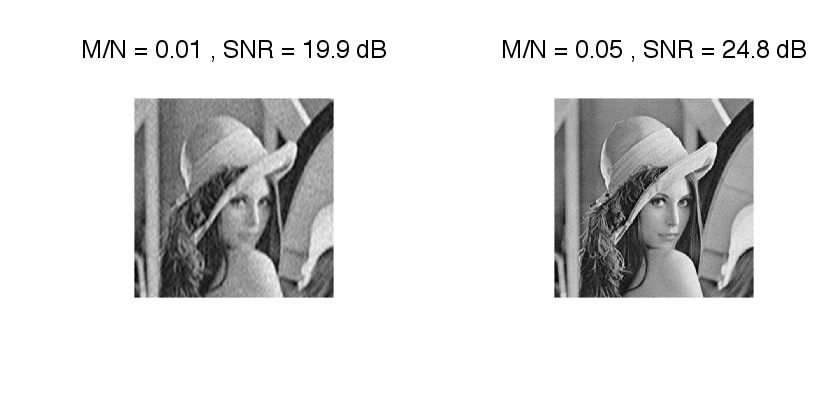In :
## Insert your code here.


Exercise 7

Compute and display in log-scale the non-linear approximation error $\epsilon[M]^2$. Compares the Fourier and DCT approximations. Store the values of $\epsilon[M]^2$ in a vector $err\_dct$.

In :
options(repr.plot.width=4, repr.plot.height=4)

source("nt_solutions/coding_1_approximation/exo7.R")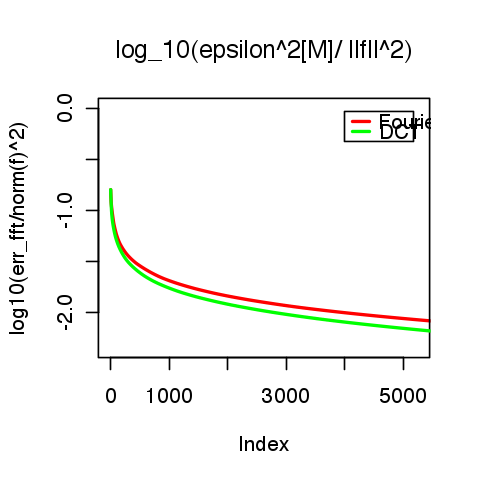In :
## Insert your code here.


## Local Cosine Approximation¶

To improve the global DCT approximation, one can approximate independantly small patches in the image. This corresponds to a decomposition in a local cosine basis, which is at the heart of the JPEG image compression standard.

The only parameter of the transform is the size of the square.

In :
w <- 16


Initialize at zero the transformed image in the local DCT basis.

In :
fL <- matrix(rep(0, length=n*n), c(n, n))


Example of patch index.

In :
i <- 5
j <- 7


For a given path index $(i,j)$, we extract a $(w,w)$ patch.

In :
P <- as.matrix(f)[((i-1)*w):(i*w-1), ((j-1)*w):(j*w-1)]


Compute the Cosine transform of the patch using the fast DCT algorithm.

In :
fL[((i-1)*w):(i*w-1), ((j-1)*w):(j*w-1)] = dct2(P)


Display the patch and its coefficients. We removed the low frequency of $P$ for display purpose only.

In :
options(repr.plot.width=7, repr.plot.height=3.5)

imageplot(P, 'Patch', c(1, 2, 1))
imageplot(dct2(P-mean(P)), 'DCT', c(1, 2, 2))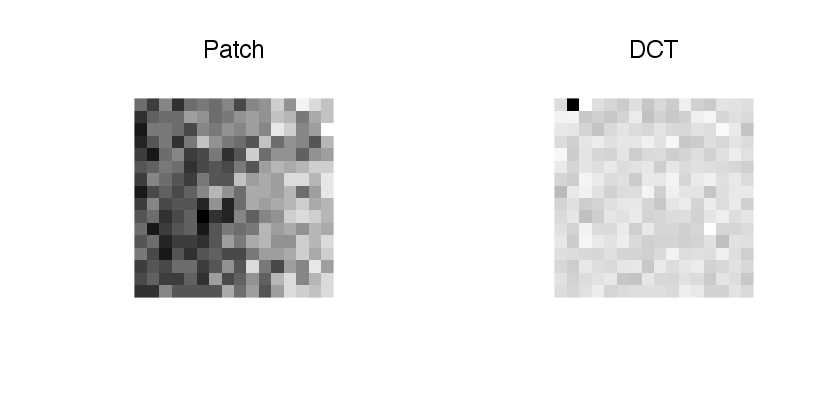Exercise 8

Compute the local DCT transform $f_L$ by transforming each patch.

In :
source("nt_solutions/coding_1_approximation/exo8.R")

In :
## Insert your code here.


Display the coefficients.

In :
options(repr.plot.width=3.5, repr.plot.height=3.5)

imageplot(clip(abs(fL),0,.005*w*w))